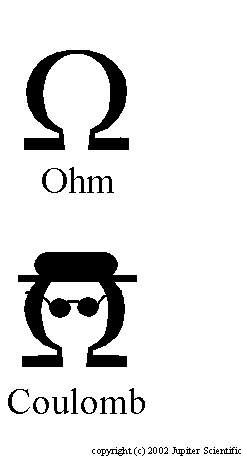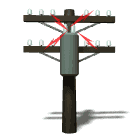APlus Physics YouTube Channel

H) Series and Parallel Circuits

UC Irvine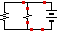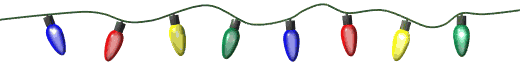Why is it a good idea to connect
Christmas lights 'in parallel'?Circuits - HTML5
Series and Parallel

Show Me The PhysicsWhich kind of circuit do we have below?

[ Flash ]

Video on Circuits

Series

1) Series Circuit - circuit that has only one current pathDorling Kindersley Books

Ex 1) Ammeter – Meter that measures Current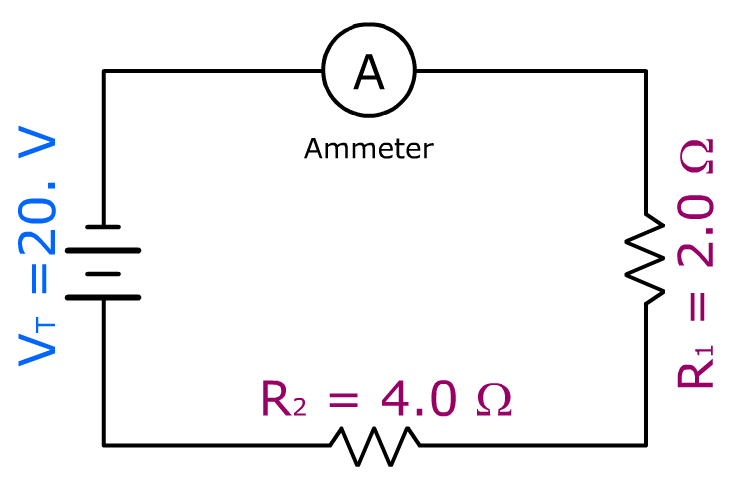Reference Table Equations

a) IT = I1 = I2 = I3 = ...

Total Current
coming from source

=

Current going
through each resistor.

b) RT = R1 + R2 + R3 + …RT = 6.0 ohms

c) VT = V1 + V2 + V3 + ……

d) VT = ITR OR  RT = VT/IT

Find total current (IT)

VT = ITRT

20. V = IT(6.0 ohms)

IT = 3.3 amps = I1 = I2

Name that Circuit

School blocks YouTube? Use the file below.